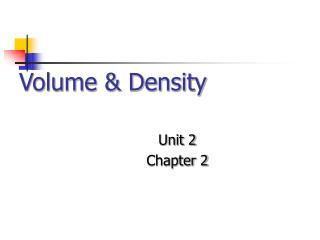DownloadDownload PresentationVolume & Density

# Volume & Density

Télécharger la présentation## Volume & Density

- - - - - - - - - - - - - - - - - - - - - - - - - - - E N D - - - - - - - - - - - - - - - - - - - - - - - - - - -
##### Presentation Transcript

1. Volume & Density Unit 2 Chapter 2

2. Volume Volume is a quantitative, extrinsic property of a substance that is usually measured indirectly. If the object is solid, there are two common methods for determining its volume. First, if the solid is geometric, we can take its measurements and then calculate the volume using a formula.

3. Geometric Formulae Rectangular Solid = lwh Cylinder = r2h Sphere = 4/3 r3 Right Prism = Area of Face * height

4. Displace This A second method for determining the volume of a solid is the Water Displacement method. In this method, you place a solid into a known volume of liquid that it does NOT dissolve in (usually water). Then, measure the change in volume.

5. Change in Volume Calculate the volume of the solid by subtraction, Vf – Vi = Vobject (final volume – initial volume = object’s volume) Some water displacement devices work by emptying the displaced liquid into a separate container where the volume can be measured directly.

6. Measuring Liquids When measuring the volume of liquids, We take advantage of the fact that liquids will conform to the shape of the container. If we have a container that is calibrated by volume, We can simply place the liquid into the container And then read the level of the liquid.

7. Size Matters Ideally, the measuring device should be as close to the size of the volume to be measured as possible. For example, you should not use a 1 liter graduated cylinder to measure 3 milliliters of liquid. Instead, use a 10 mL graduated cylinder.

8. Big No-No Never use a beaker or an Erlenmeyer flask to measure the volume of a liquid!

9. Reading Liquids When a liquid is placed into a narrow tube, A meniscus will form due to the Liquid’s attraction to (or repulsion from) the tube. It is important that you read the volume of the liquid correctly.

10. Reading Menisci With a concave meniscus, read the level at the bottom of the meniscus. Read Here!!!

11. Reading Menisci With a convex meniscus, read the level at the top of the meniscus. Read Here!!!

12. Gases Since gases fill the entire volume of the container, The volume of the container is the volume of the gas; However, we have to take into account the temperature and pressure because they affect gases so much. We will discuss gas volumes in a later unit.

13. Density Density is a quantitative, intrinsic property that is derived from two extrinsic properties: mass and volume. Density is a measure of how tightly packed the matter is inside of an object. It is calculated by dividing the mass by the volume.

14. Density II It does not depend on the shape of the object or how much is present – remember, density is an intrinsic property. Because of this, we can use densities to identify substances (unlike mass or volume).

15. Density III The formula for density is: d = m / v

16. Rearranging It If you are given density & volume, you can rearrange the formula to determine the mass: m = d * v If you are given density & mass, you can rearrange the formula to determine the volume: v = m / d